<h1>定义</h1>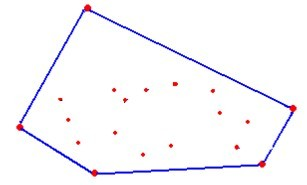<h1>前置芝士</h1>

<h2>计算集合中的相等</h2>

const double EPS = 1e-9; // or smaller than
inline bool equ(double x,double y){
return std::fabs(x-y) < EPS;
}


<h2>向量</h2>

<h3>定义</h3>

$\vec{AB}$ 同时也可以使用 $(^{x_A−x_B}_{y_A−y_B})$ 来表示。

struct Point{ // 为了方便使用点和向量混合定义的方式
double x,y;

Point() {}
Point(double x,double y) : x(x),y(y) {}
}


<h3>向量的模</h3>

double len(){
return std::sqrt(xx+yy);
}


<h3>向量的运算</h3>

Point operator - (const Point &t) const {
return Point(x-t.x,y-t.y);
}


<h3>向量的点积</h3>

$a \cdot b$ 的几何意义是 $a$ 在 $b$ 上的投影长度乘上 $b$ 的模长。
$a\cdot b=|a||b| \cos \theta$ ，其中 $\theta$ 是一个区分正负的夹角。

$$a⋅b=x_1y_1+x_2y_2$$

<h4>基本运用</h4>

<h3>二维叉积</h3>

double operator * (const Point &t) const {
return xt.y - yt.x;
}


<h4>基本应用</h4>

<h2>极坐标系</h2>

<h3>定义</h3>

<h3>极角排序</h3>

bool cmp(const Point &a,const Point &b){
return std::fabs((a-p)(b-p)) < EPS ? (a-p).len() < (b-p).len() : (a-p)(b-p) > 0;
}


<h1>Graham扫描法</h1>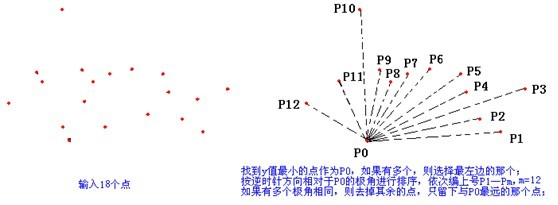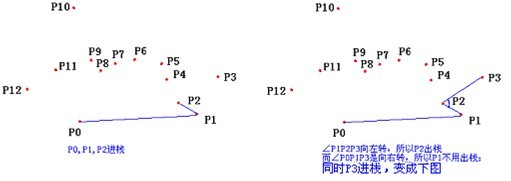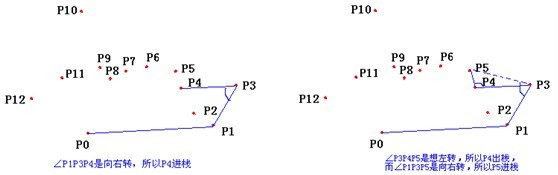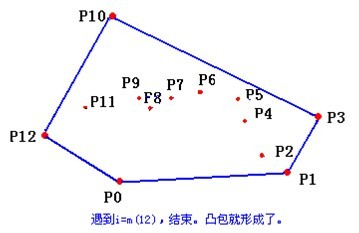#include <algorithm>
#include <iostream>
#include <cstring>
#include <climits>
#include <cstdio>
#include <vector>
#include <cmath>
#include <queue>
#include <stack>
#include <map>
#include <set>

#define Re register
#define LL long long
#define U unsigned
#define FOR(i,a,b) for(Re int i = a;i <= b;++i)
#define ROF(i,a,b) for(Re int i = a;i >= b;--i)
#define SFOR(i,a,b,c) for(Re int i = a;i <= b;i+=c)
#define SROF(i,a,b,c) for(Re int i = a;i >= b;i-=c)
#define CLR(i,a) memset(i,a,sizeof(i))
#define BR printf("--------------------n")
#define DEBUG(x) std::cerr << #x << '=' << x << std::endl

const int MAXN = 200000+5;
const double EPS = 1e-9;

inline bool equ(double x,double y){
return std::fabs(x-y) < EPS;
}

struct Point{
double x,y;

Point() {}
Point(double x,double y) : x(x),y(y) {}

Point operator - (const Point &t) const { // 点减法
return Point(x-t.x,y-t.y);
}

double operator * (const Point &t) const { // 叉积
return xt.y - yt.x;
}

bool operator < (const Point &t) const { // 找出极点
return equ(x,t.x) ? y < t.y : x < t.x;
}

bool operator == (const Point &t) const { // 判断同一个点
return equ(x,t.x) && equ(y,t.y);
}

double len(){ // 模长
return std::sqrt(xx+yy);
}
}p[MAXN],S[MAXN];

int top;

bool cmp(const Point &a,const Point &b){
return std::fabs((a-p)(b-p)) < EPS ? (a-p).len() < (b-p).len() : (a-p)(b-p) > 0;
// 同一直线比较长度否则比较谁在右侧
}

int N;

int main(){
scanf("%d",&N);
FOR(i,1,N) scanf("%d%d",&p[i].x,&p[i].y);
std::sort(p+1,p+N+1); // 最小的 p
std::sort(p+2,p+N+1,cmp); // 极角排序
N = std::unique(p+1,p+N+1)-(p+1);
FOR(i,1,N){
while(top > 1 && (p[i]-S[top-1])*(S[top-1]-S[top-2]) > 0) // 这个点在逆时针方向弹掉
top--;
S[top++] = p[i];
}
double ans = 0.0;
FOR(i,0,top-1){
ans += S[i]*S[(i+1)%top];
}
printf("%.5f",ans);
return 0;
}


Last modification：April 7th, 2020 at 10:14 pm